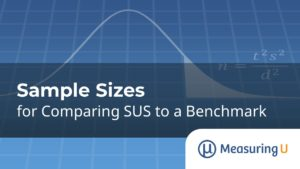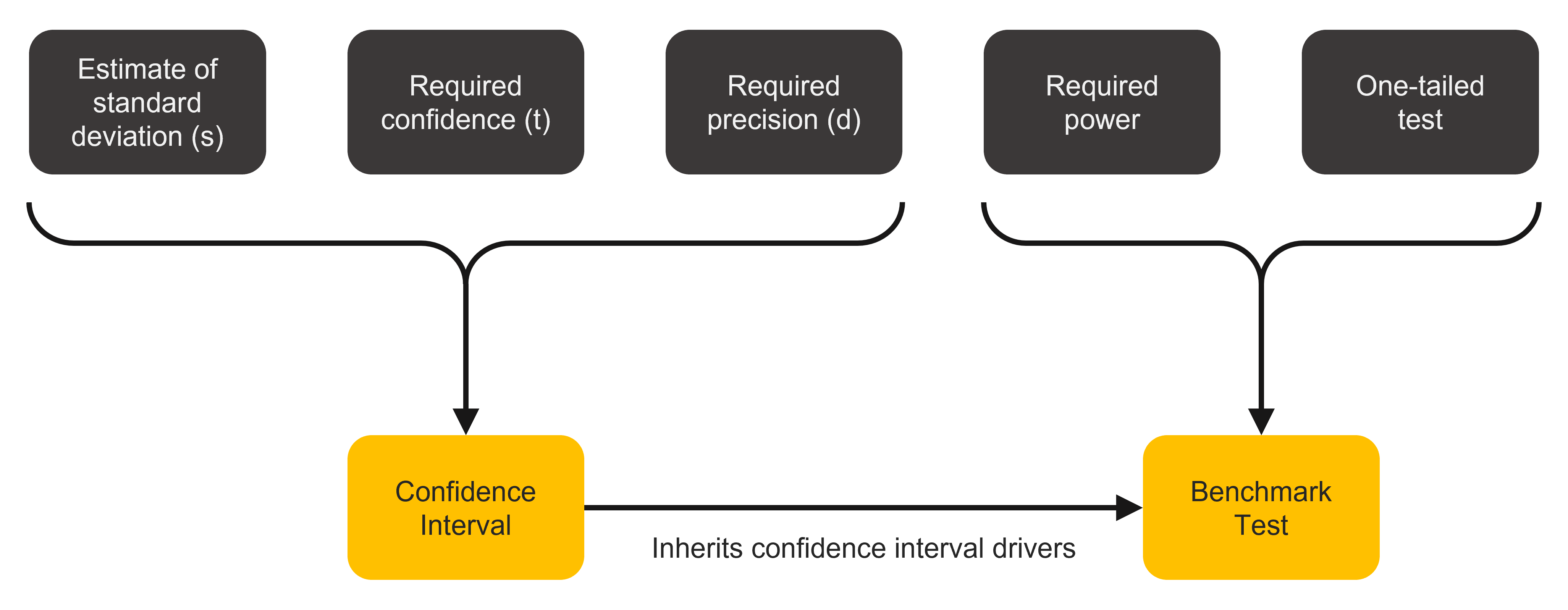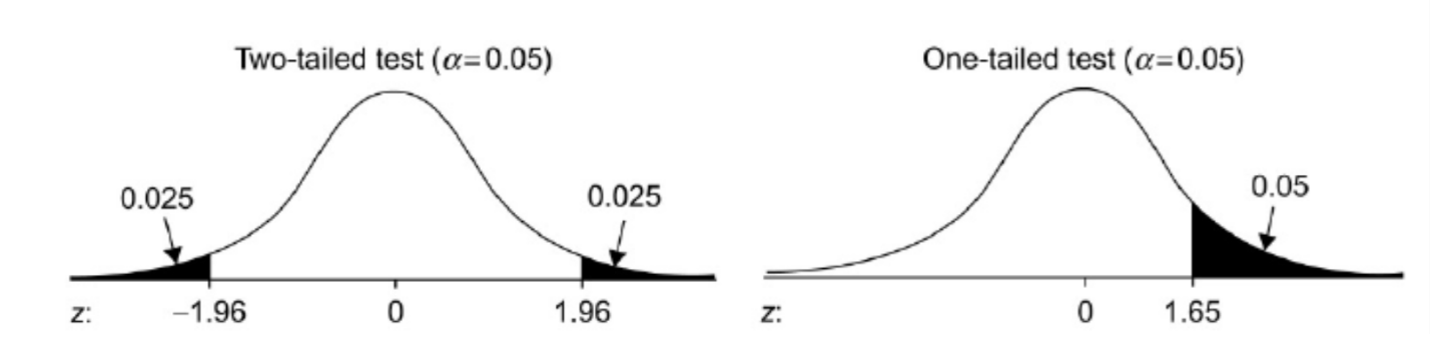# Sample Sizes for Comparing SUS to a Benchmark

Jim Lewis, PhD and Jeff Sauro, PhDThe System Usability Scale (SUS) has been used in industrial user experience research since the mid-1980s. Despite its age, the SUS is still a popular measure, widely used in benchmark tests of software products to measure perceived usability. One reason for its popularity is the extent to which its measurement properties have been comprehensively studied over the past two decades, ensuring those properties are increasingly well understood.

Thanks to the wide usage of the SUS, everyone is familiar with its scoring system (from 0 to 100) and its known benchmark scores: below 50 is pretty horrible, 68 is about average, and above 80 is pretty darn good (just above the boundary between B+ and A− in our curved grading scale for the SUS).

More precise benchmark scores are also available from published studies and industry reports. For example, we regularly collect SUS scores for dozens of consumer and business products in our UX benchmark reports. Our 2020 benchmark report reported SUS scores on dozens of products, including Microsoft Word, which had a SUS score of about 75 (74.7 ± 3.5—see Figure 1). That puts Word’s perceived usability above the average score of 68 but below the well-above-average score of 80.Figure 1: MeasuringU® 2020 benchmark SUS for Microsoft Word (95% margin of error: ±3.5).

In that report, we computed the SUS score from 111 current users’ ratings of MS Word. What sample size is needed to know if Word (or any product) achieves, exceeds, or falls below a benchmark?

We covered in an earlier article how to find the sample size needed to generate a specified margin of error/confidence interval, such as ±5 points. But what sample size do you need to compare a SUS mean to a SUS benchmark?

In this article, we discuss the somewhat more complex sample size estimation method required to compare a mean SUS with a set benchmark, like the typical average of 68 or the well-above-average score of 80.

## What Drives Sample Size Requirements for Benchmark Tests?

Not to be intentionally confusing, but we’ll often refer to “benchmark testing” to mean a few things. First, it’s used loosely to refer to the process of collecting metrics (e.g., benchmarking), which we cover extensively in Benchmarking the User Experience.

It also refers to how metrics collected within a study will be used. They can be used to establish the current experience of a product (a new benchmark) in which you would use confidence intervals around the benchmark.

Metrics can be used to compare against a prior experience or a competitive experience (comparative or competitive). Metrics can also be used to compare against established thresholds (a benchmark). We’re focusing on this final case, where a sample of SUS scores is collected, and then the mean is compared to a set benchmark value.

The historical SUS mean of 68 is commonly used as a benchmark to test when a SUS mean is above average. For testing to see if a product has levels of perceived usability that are well above average, it’s common to set the benchmark to 80.

As shown in Figure 2, you need to know five things to compute the sample size before comparing scores to a benchmark. The first three are the same elements required to compute the sample size for a confidence interval (for a comprehensive discussion of these three elements, see our previous article).

1. An estimate of the SUS standard deviation: s
2. The level of confidence (typically 90% or 95%): t
3. The desired margin of error around your estimate (using points or percentages): d

Sample size estimation for benchmark tests requires two additional considerations:

1. The power of the test
2. The level of confidence for a one-sided (one-tailed test)Figure 2: Drivers of sample size estimation for benchmark comparisons.

### Power

The power of a test refers to its capability to detect a difference between observed measurements and hypothesized values when there really is a significant difference. The power of a test is not an issue when you’re just estimating the value of a parameter, but it matters when testing a hypothesis. Analogous to setting the confidence level to 1 − α (the acceptable level for Type I errors, or false positives), power is 1 − β (the acceptable level for Type II errors, or false negatives).

### One-tailed testing

Most statistical comparisons use a strategy known as two-tailed testing. The term “two-tailed” refers to the tails of the distribution of the differences between the two values. The left distribution in Figure 3 illustrates a two-tailed test showing the rejection criterion (α = .05) evenly split between the two tails.Figure 3: Two- and one-sided rejection regions for two- and one-sided significance tests.

For most comparisons, two-tailed tests are appropriate. However, when you test an estimated value against a benchmark, you care only that your estimate is significantly better than the benchmark. When that’s the case, you can conduct a one-tailed test, illustrated by the right distribution in Figure 3. Instead of splitting the rejection region between two tails, it’s all in one tail. The practical consequence is that the bar for declaring significance is lower for a one-tailed test.

The area in one tail for a two-sided test with α = .10 is the same as a one-sided test with α = .05. This factor decreases the sample size relative to computing a two-tailed confidence interval.

### Putting the Values Together

With the five ingredients ready, we use the same formula we used for sample sizes for confidence intervals:Here, s is the standard deviation (s2 is the variance), t is the t-value for the desired level of confidence AND power, and d is the targeted size for the interval’s margin of error (i.e., precision).

The difference compared to the confidence interval computation is that t is actually the sum of two t-values, one for α (related to confidence) and one for β (related to power, always one-sided). For a 90% confidence level and 80% power this works out to be about 1.645 + 0.842 = 2.5.

When you don’t need more power, the default power level is 50%, at which t for power = 0, making it the same result as a confidence interval. Any larger value for power (commonly 80%) makes the value of t larger, which will increase the estimated sample size.

Consider the inclusion of power in sample size estimation as an insurance policy. You purchase a policy by increasing your sample size to increase your likelihood of finding statistically significant results if the standard deviation is a little higher than expected or the observed value of d is a bit lower.

Table 1 shows the sample sizes required for two levels of confidence (90 and 95%), one level of power (80%), and various levels of d from 1 to 15 (where d is the minimum amount by which we need to significantly beat the benchmark). This table uses our best estimate of the typical standard deviation of the SUS (17.7).

dSUS; 90%SUS; 95%
15811
101621
7.52736
55880
2.5228312
2355486
11,4141,939

Table 1: Sample size requirements for various SUS benchmark tests (one-tailed, 80% power, s = 17.7).

For example, to declare that you have significantly beaten a SUS benchmark of 70 with 90% confidence, 80% power, and a critical difference of 15, you will need a sample size of eight—but you will also need the observed SUS mean to be 85 (70 + 15) or higher.

At the other end of the table, if you have the same benchmark (70), 95% confidence, 80% power, and a critical difference of 1, you’ll only need the observed SUS mean to be 71 (70 + 1), but you’ll need a sample size of 1,939.

In this table, there’s a sort of Goldilocks zone for reasonably small requirements for the critical difference (d from 2.5 to 5), which have reasonably attainable sample size requirements (n from 58 to 312).

The table also shows how sample size estimates balance statistics and logistics. The math for a high level of precision may indicate aiming for a sample size of 1,000, but the feasibility (cost and time) of obtaining that many participants might be prohibitive, even in a retrospective survey or unmoderated usability study.

## What about the Different Estimates for the Standard Deviation of SUS?

As documented in A Practical Guide to the System Usability Scale, estimates of the standard deviation of the SUS from different sources ranged from 16.8 to 22.5. A standard deviation of 17.7 is typical, but what if your standard deviation is larger than that?

For example, suppose your estimate is 20. Because this estimate is larger than the 17.7 used to build Table 1, when all other things are the same (e.g., confidence and precision levels) you’ll need larger sample sizes, as shown in Table 2.

dSUS; 90%SUS; 95%
151013
102027
7.53446
574101
2.5290398
2452620
11,8052,475

Table 2: Sample size requirements for various SUS benchmark tests (one-tailed, 80% power, s = 20).

If your SUS data has a standard deviation close to 17.7, use Table 1. If it’s closer to 20, use Table 2. If it’s in between, then interpolate the values in Tables 1 and 2 to get an idea about the approximate sample size. If you need a more precise estimate, see the following technical note.

Technical Note: If you’re working with SUS data with a very different standard deviation from 17.7 or 20, you can do a quick computation to adjust the values in these tables. The first step is to compute a multiplier by dividing the new target variance (s2) by the variance used to create the table. Then multiply the tabled value of n by the multiplier and round it off to get the revised estimate. For example, if the target standard deviation (s) is 19.1, the target variability (s2) is 364.81. The variability from Table 2 is 400 (202), making the multiplier 364.81/400 = .912. To use this multiplier to adjust the sample size of 398 for 95% confidence and critical difference of 2.5 shown in Table 2, multiply 398 by .912 and then round it off to 363.

## Summary and Takeaways

What sample size do you need when conducting a SUS benchmark test? To answer that question, you need several types of information, some common to all sample size estimation (confidence level to establish control of Type I errors, standard deviation, and margin of error or critical difference) and others unique to statistical hypothesis testing (one- vs. two-tailed testing, setting a level of power to control Type II errors).

We provided two tables based on a typical standard deviation for the SUS in retrospective UX studies (s = 17.7) and a more conservative standard deviation (s = 20).

For UX researchers working in contexts where the typical standard deviation of the SUS might be different, we provided a simple way to increase or decrease the tabled sample sizes for larger or smaller standard deviations.

0
0